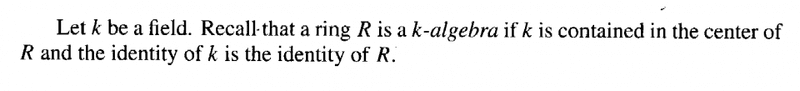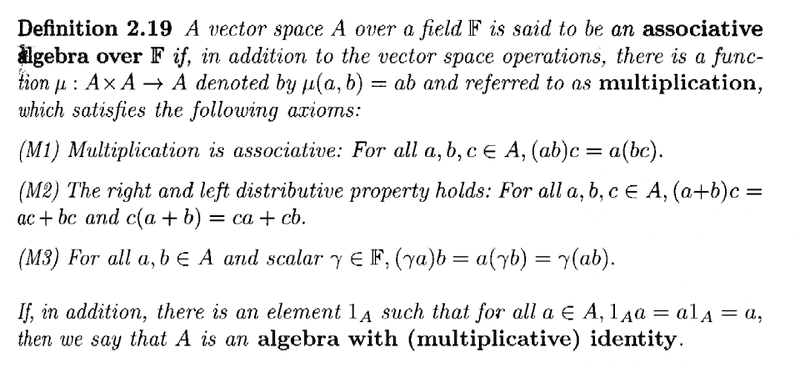# K-algebra/associative algebra - equivalence of definitions

Gold Member
I am reading Dummit and Foote's book "Abstract Algebra" (3rd Edition) and am focused on Chapter 15: Commutative Rings and Algebraic Geometry ... ...

On page 657 D&F give a definition of a k-algebra ... as follows:I have to say I do not find that this definition gives me a good intuitive idea of the nature of an algebra over a field ... ...

I much prefer the definition given by Cooperstein in his book "Advanced Linear Algebra" (Second Edition) where he defines what he calls an "associative algebra over a field F" (which I take to be the same as D&F's k-algebra ... ... is that right?) ... ...

Cooperstein's definition is as follows:I find Cooperstein's definition more clear regarding the nature of an algebra ... ... but I am currently working from D&F and wish to fully understand the approach to an algebra ... but I am unclear on exactly why these two definitions are the same or equivalent ... ...

Can someone please help me to formally and rigorously prove that these two definitions are equivalent ... ...

Help will be much appreciated ...

Peter

#### Attachments

fresh_42
Mentor
2021 Award
The difference is only in the point of view. D&F starts with a ring, i.e. there is a group addition and a sort of multiplication.
In order to turn it into an algebra, there must be a vector space. Addition is already fine. So there's only to define the scalar multiplication.
First, you have to have a field ##k## anywhere. Since there is only the ring, it has to be part of it, i.e. a subset. A field is commutative, so ##k## has to be in the center of the ring. Because we still are in the ring, there can only be one element ##1,## so it has to be the same in the ring and the field. This is the way a ring can be considered as a ##k-##algebra (sometimes).

The two definitions, however, are not the same or even equivalent. Neither need a ring contain a field, e.g. ##\mathbb{Z}##, nor has an algebra to be a ring, because the scalar field of an algebra is usually not part of it. Algebras simply have a multiplication among its elements. They need not to be associative, e.g. Lie algebras, they don't automatically have a ##1##, e.g. ##\mathbb{R}^3## with the cross-product, and they don't have to be commutative.
Ok, a ring doesn't have to be one of these either, but often they do. In general can be said:

An algebra is a vector space where a bilinear multiplication among its elements is defined. It always has scalar multiplication.
A Ring is an additive group, in which a distributive multiplication is defined. There is generally no scalar field contained.
An extreme case is a field. It is by itself a ring, as well as a one-dimensional algebra; even if a boring one.

Edit: Both concepts share similar properties, and there exist examples and constructions to, e.g. apply an external field as scalar domain to a ring. In general both concepts simply refer to different approaches and areas of research.

•Math Amateur
micromass
Staff Emeritus
Homework Helper
The two definitions are equivalent for unital associative rings. You should try a proof yourself. Here is a very rough sketch:

- Given the D&F definition where ##k\subseteq R##, define a scalar multiplication as ##k\times R\rightarrow R:(\alpha,r) \rightarrow \alpha r## the usual multiplication and check all the properties.

- Given the Cooperstein definition where you have a scalar multiplication ##k\times R\rightarrow R##, then define the injection ##k\rightarrow R: \alpha\rightarrow \alpha\cdot 1##.

•Math Amateur
mathwonk
Homework Helper
not trying to be more precise, just an intuitive approach. A ring, you know what that is. An algebra is essentially just two rings, one inside the other, at least in the commutative case. So in my commutative algebra course, an algebra was defined as just a ring map, A-->B. Of course for us all rings maps took 1 to 1. So we did not worry about the ring A being actually inside B, and the map could be maybe not injective.

The point is have one ring B, and also the ability to multiply elements of B by the elements of another ring A. But you want that multiplication to behave well, like scalar multiplication in a vector space, so you make the identities agree and you make A live, or map, inside the center of B, i.e. elements of A commute with elements of B.

To make a long story short, the basic example of an algebra is a ring B together with the center of B, called A. Or more generally it is a ring B and a (unital) map from a ring A to the center of B. (I always think of my rings as associative and having a unit. A rng (ring without unit) can always be enlarged to have one.)

It may be helpful to look at some examples, such a matrix algebras, division algebras, polynomial algebras, function algebras, C* algebras...maybe R^3 with cross product.

•Math Amateur
mathwonk
Homework Helper
notice you are reading a chapter on algebraic geometry. all rings in chapter 15 of DF are commutative, so their definition of a k algebra in that setting is merely a ring that contains k. on the n ext page they even prove that every finitely generated k alkgebra in that case is simply a quotient of a polynomial ring k[X1,....,Xn]. That's it. how could it be simpler?

The point is in that chapter, one is studying algebraic sets defined over a given field,, so the field k is the "ground field" or coefficient field, and the ring R is the ring of polynomial functions on the algebraic set being studied. Recall that an algebra is merely a relative version of a ring, i.e. it invoklves two rings relatd by a map. In algebraic geometry we distinguish betwen points and sets of those points. The ring of a point is the field k, since all functions on a point are constants, and the ring of the point set is the ring R.

Onecan jaz up the idea of an algebra here to mean B is an A algebra where both A and B are k algebras. Then it means we have a pair of algebraic sets, with function rings A and B, and a map from one set to the other, so that the induced map of pulling back functions is the k algebra map A-->B. This is a k algebra map since constant functions pull back to constant functions.

•Math Amateur
fresh_42
Mentor
2021 Award
@Math Amateur

Hi Peter,

it is important to get used to polynomial rings and the concept of ideals and rings like integral domains, quotient rings and so on before you start deepening algebraic geometry. This is an area which IMO isn't very helpful to start with. It's far easier to understand if you are firm in algebraic structures like groups, rings, fields and eventually algebras.

However, algebras are so general that they cover many very different types and sorts of multiplications. You won't need to know them all in detail. They usually occur naturally when one is working on mathematical applications: tensor-, Graßmann-, Lie-, Clifford-, Heisenberg-, Poincaré-Algebras in physics, Boolean Algebras in logic and computer science, σ-Algebras in measure theory, heredity algebras in biology and probably many more. The reasonable multiplication of mappings and functions is enough to know at the beginning. (@ all: answers are senseless I'm on the run ...)

It has been a good idea of @micromass to prove the equivalence of the two definitions above in the special case he has mentioned as an exercise. (It's mainly an exercise to distinguish carefully the different concepts.)

•Math Amateur
mathwonk
Homework Helper
in mathematics we give definitions to capturw the properties of objects we want to study again and again. so we are motivatred by the example we are interested in and we look for its crucial properties. an algebra thus has many possible definitions depending on which ones one is intertested in. I personally suggest it is not useful to get distracted by wondering what the most general definiton of an "algebra" is if you are really trying to read chapter 15 of DF. In thnat cha\pter the only algebras involved are just polynomial algebras over a field of coefficients, and quotients of these. So if you are familiar with polynomial rings then I would just move on and read more of the chapter.

E.g. choose an irreducible polynomial f(X,Y) in two variables with complex coefficients and consider the plane curve C in C^2 where this polynom ial vanishes. This isn algebraic plane curve. Now consider two polynomials g,h, in C[X,Y] to be equivalent if they have the same values at every point of the curve C. This defines an equivalence relation on C[X,Y] where two polynomials are euivalent preciwely when their difference g-h is divisible by f. I.e. the equivalence classes make up the quotient ring C[X,Y]/(f).

This quotient ring is called the ring of polynomial functions on the curve C. In partocular this is a C algebra. These are the only sort of algebras that will be discussed in this chapter. I.e. in general they consider a finite set of polynomials, f1,...,fr in k[X1,...,Xn] and look at the set in k^n where all r of these polynomials vanish. This is an algebraic set V, called the zero locus of the polynomials f1,...,fr. Then again al p[olynomial in k[X1,...,Xn] define polynom ial functions on V and two p[oplynomials define the same function on V if their difference bel;ongs to the ideal (f1,...,fr) generated by the fj's. But in fact this is not quite the compklete story. If their difference belongs even to the "radical" of this ideal they will also define the same function. So this theorem, the so called Hilbert n ull stellensatz, is a basic result.

If we had SSUMED THE IDEAL THEY GENERATE WAS PRIME, we would have been in a situation analogous to the first case whn we asumed the polynomialf was irreducible and we would not have had to wiorry about this. anyway. I would not waste timemover the definition of n algebra but get on with learning somethign ab out the specific algebras of interest in this beautiful surbject, namely polynomial algebras.

•Math Amateur
Gold Member
Thanks to fresh_42, micromass and mathwonk... for the thoughtful and helpful posts ... read them all at least twice!

Thanks mathwonk for the strategy to approach Ch 15 ...

Peter